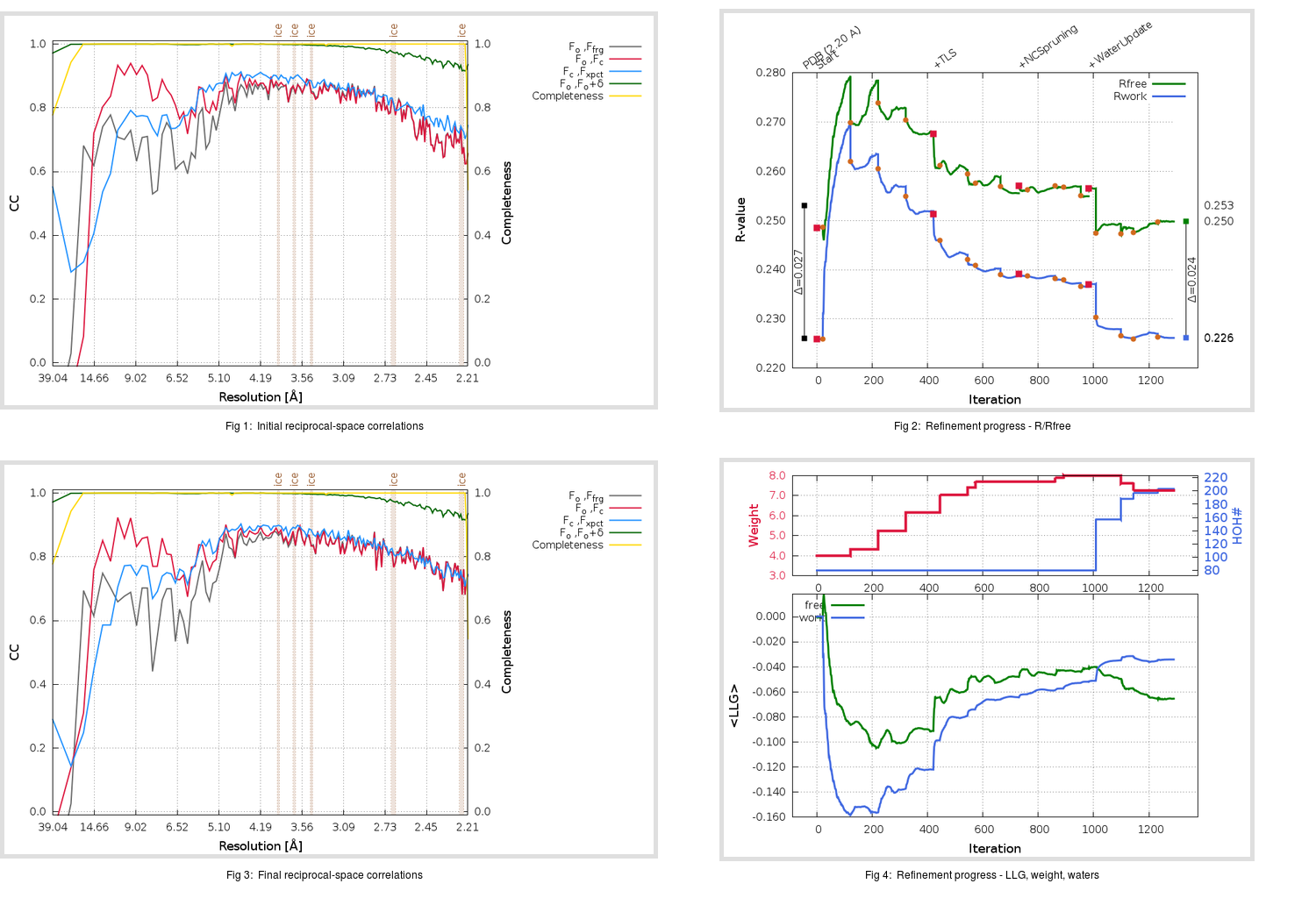Content:

```    Diffraction limits & principal axes of ellipsoid fitted to diffraction cut-off surface:
2.154         1.0000   0.0000   0.0000       0.964 a* - 0.267 c*
2.175         0.0000   1.0000   0.0000       b*
2.165         0.0000   0.0000   1.0000       c*
```

## Deposited

` `
 Date deposited Date data collection Resolution R, Rfree 20030814 20030716 2.20 0.2260 0.2530

Molprobity (CCP4 7.0 version) summary:

```Ramachandran outliers =   2.67 %
favored =  91.00 %
Rotamer outliers      =  11.78 %
C-beta deviations     =     5
Clashscore            =  19.03
RMS(bonds)            =   0.0207
RMS(angles)           =   2.32
MolProbity score      =   3.11
Resolution            =   2.20
R-work                =   0.2260
R-free                =   0.2530
```

```Number of waters      =    80

<B> (all atoms) =   34.45 ( sd =   16.85 ) for       4752 non-hydrogen atoms
<B>   (protein) =   34.45 ( sd =   16.92 ) for       4672 non-hydrogen atoms
<B>     (water) =   34.59 ( sd =   12.05 ) for         80 non-hydrogen atoms
<B>    (others) =    0.00 ( sd =    0.00 ) for          0 non-hydrogen atoms

B min/max       (all non-hydrogen atoms) =    5.14 /   98.83
B min/max   (protein non-hydrogen atoms) =    5.14 /   98.83
B min/max     (water non-hydrogen atoms) =   14.22 /   65.27
B min/max     (other non-hydrogen atoms) =    0.00 /    0.00
```

## BUSTER (re-)refinement

` `

Molprobity (CCP4 7.0 version) summary:

```Ramachandran outliers =   0.50 %
favored =  94.83 %
Rotamer outliers      =   9.07 %
C-beta deviations     =     3
Clashscore            =   4.65
RMS(bonds)            =   0.0118
RMS(angles)           =   1.60
MolProbity score      =   2.32
Resolution            =   2.21
R-work                =   0.2260
R-free                =   0.2497
```

```Number of waters      =   203

<B> (all atoms) =   36.11 ( sd =   15.01 ) for       4875 non-hydrogen atoms
<B>   (protein) =   35.91 ( sd =   14.59 ) for       4672 non-hydrogen atoms
<B>     (water) =   40.64 ( sd =   22.24 ) for        203 non-hydrogen atoms
<B>    (others) =    0.00 ( sd =    0.00 ) for          0 non-hydrogen atoms

B min/max       (all non-hydrogen atoms) =    9.03 /  300.00
B min/max   (protein non-hydrogen atoms) =   13.41 /   94.25
B min/max     (water non-hydrogen atoms) =    9.03 /  300.00
B min/max     (other non-hydrogen atoms) =    0.00 /    0.00
```

Refinement progression:Results:

` `
 File Remark 1UK2_aB_refine.01_04_refine.pdb.gz exact refinement commands are in header 1UK2_aB_refine.01_04_refine.mtz.gz including original deposited data and several re-refinement map coefficients 1UK2_aB_refine.01_04_BUSTER_model.cif.gz including any non-standard compound restraints 1UK2_aB_refine.01_04_BUSTER_refln.cif.gz